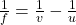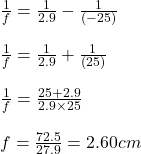## brainly A person’s eye lens is 2.9 cm away from the retina. This lens has a near point of 25 cm and a far point at infinity. What must the f

Question

brainly A person’s eye lens is 2.9 cm away from the retina. This lens has a near point of 25 cm and a far point at infinity. What must the focal length of this lens be in order for an object placed at the near point of the eye to focus on the retina

in progress 0
7 months 2021-07-16T08:50:37+00:00 1 Answers 3 views 0

1. Answer: The focal length of the lens is 2.60 cm

Explanation:

The equation for lens formula follows:where,

f = focal length = ? cm

v = image distance = 2.9 cm

u = Object distance = -25 cm

Putting values in above equation, we get:Hence, the focal length of the lens is 2.60 cm